Finding sum of n terms

Chapter 5 Class 10 Arithmetic Progressions (Term 2)
Concept wise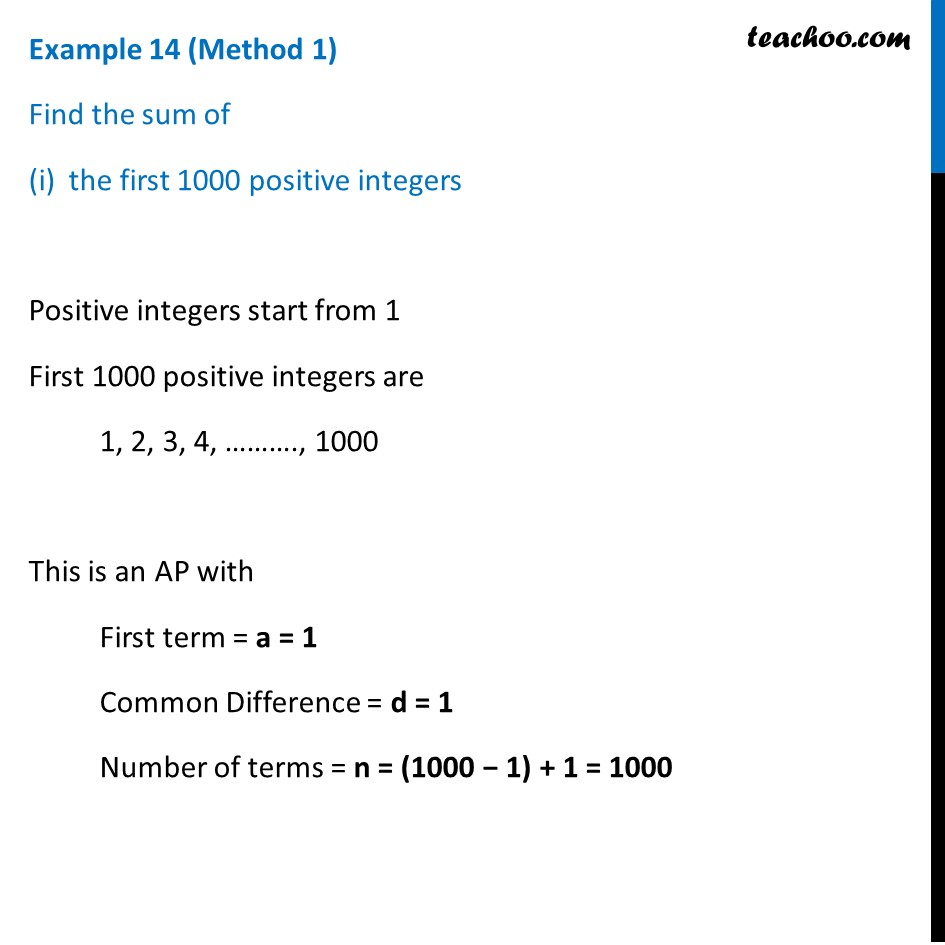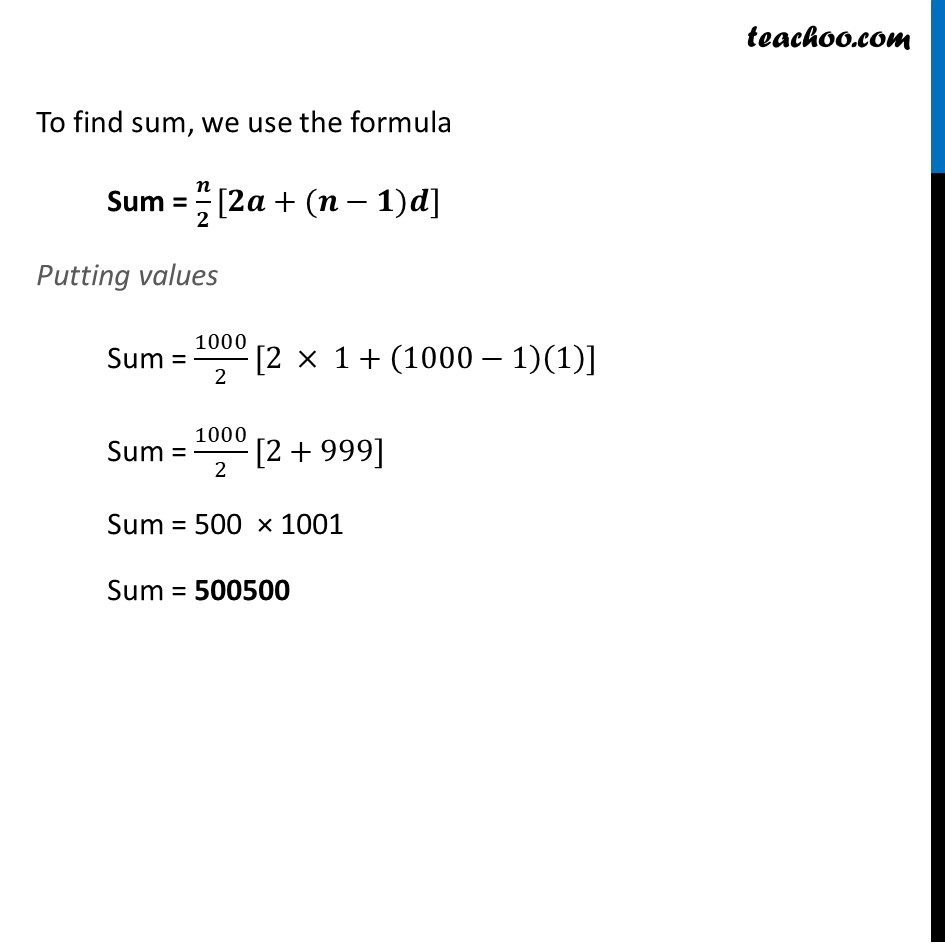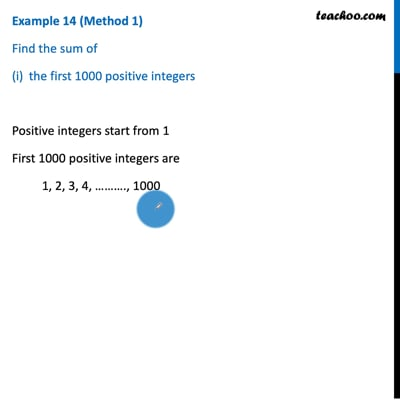This video is only available for Teachoo black users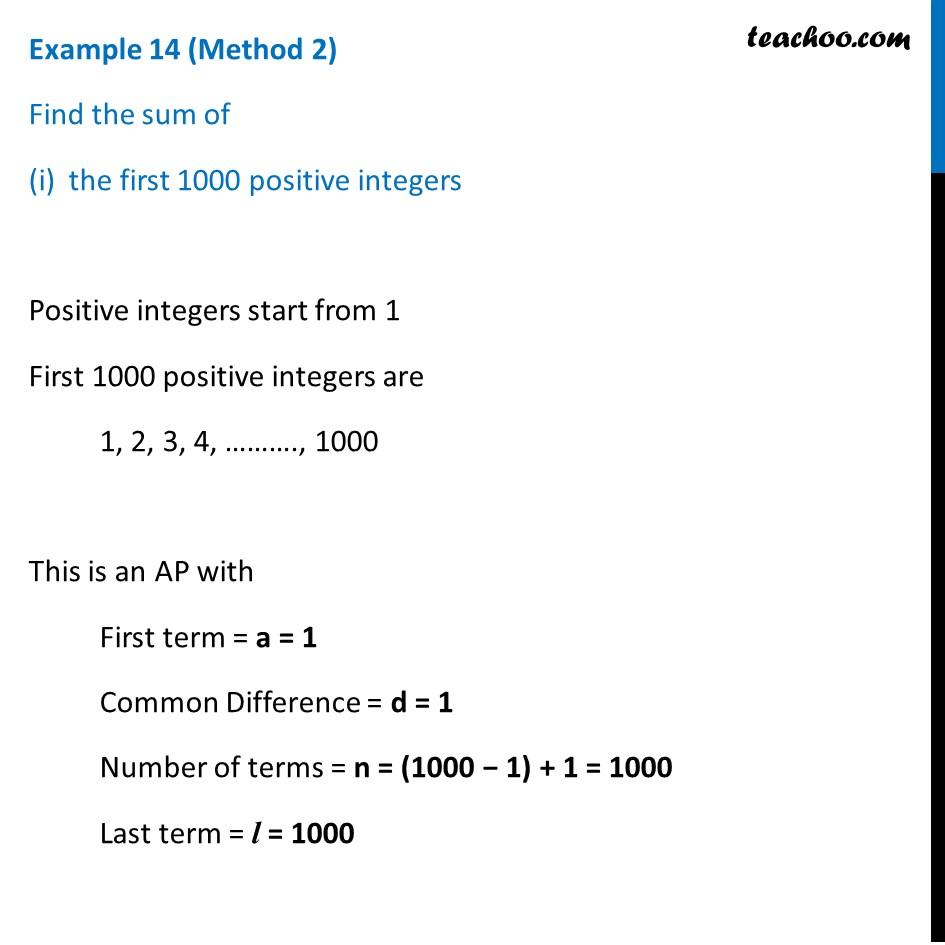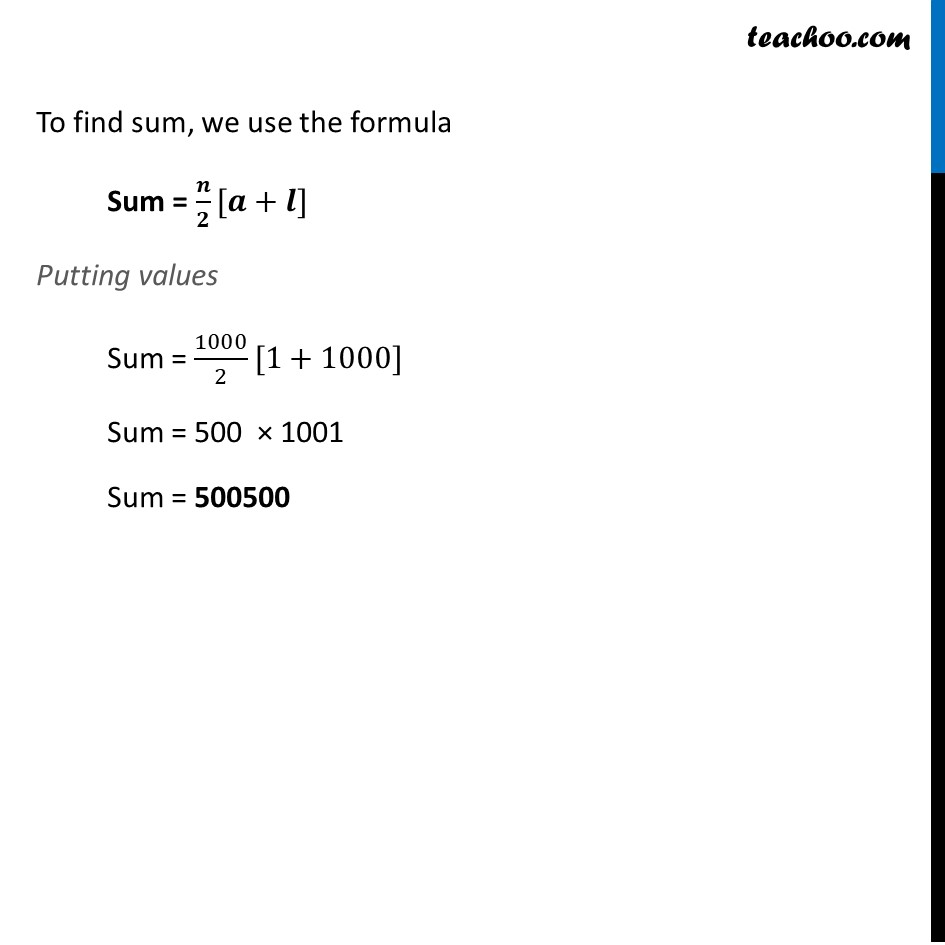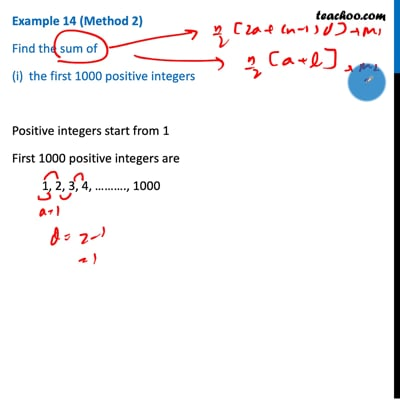This video is only available for Teachoo black users

### Transcript

Example 14 (Method 1) Find the sum of the first 1000 positive integers Positive integers start from 1 First 1000 positive integers are 1, 2, 3, 4, ………., 1000 This is an AP with First term = a = 1 Common Difference = d = 1 Number of terms = n = (1000 − 1) + 1 = 1000 To find sum, we use the formula Sum = 𝒏/𝟐[𝟐𝒂+(𝒏−𝟏)𝒅] Putting values Sum = 1000/2[2 × 1+(1000−1)(1)] Sum = 1000/2[2+999] Sum = 500 × 1001 Sum = 500500 Example 14 (Method 2) Find the sum of the first 1000 positive integers Positive integers start from 1 First 1000 positive integers are 1, 2, 3, 4, ………., 1000 This is an AP with First term = a = 1 Common Difference = d = 1 Number of terms = n = (1000 − 1) + 1 = 1000 Last term = l = 1000 To find sum, we use the formula Sum = 𝒏/𝟐[𝒂+𝒍] Putting values Sum = 1000/2[1+1000] Sum = 500 × 1001 Sum = 500500